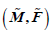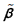# 一类Finsler子流形的研究The Study of a Class of Finsler Submanifolds

DOI: 10.12677/PM.2021.116116, PDF, HTML, XML, 下载: 12  浏览: 78  科研立项经费支持

Abstract: In this paper, we mainly use a natural identity and consider a class of special manifoldswith an  (α,β)-metricin which ã is the Riemannian metric, andis a one-form. We aim to study a class of special manifolds by using the natural identity. Under certain conditions, there are no closed orientable BH-minimal submanifolds and closed orientable HT-minimal surfaces.

1. 引言

1972年，M. Matsumoto  推广了Randers度量的概念，得到了 $\left(\alpha ,\beta \right)$ -度量。 $\left(\alpha ,\beta \right)$ -度量

$F=\alpha \varphi \left(\beta /\alpha \right)$ 是由Riemann度量 $\alpha =\sqrt{{a}_{ij}\left(x\right){y}^{i}{y}^{j}}$ 和1-形式 $\beta ={b}_{i}\left(x\right){y}^{i}$ 构成的一类重要的Finsler度量，其中 $\varphi \left(s\right)$ 是定义在开区间 $\left(-{b}_{0},{b}_{0}\right)$ 上的光滑正函数，并满足 $\varphi \left(0\right)=1$ 使得 $F$ 为正定的Finsler度量。不难看出，这类 $\left(\alpha ,\beta \right)$ -度量包含了所有的Riemann度量( $\varphi =1$ 或者 $\beta =0$ )，这是Finsler几何中一类重要的度量，它们已经被应用到物理、生物等学科  。因此，人们对这类特殊的度量进行了深入研究。当 $\varphi =1+s$ 时， $\left(\alpha ,\beta \right)$ -度量 $F=\alpha +\beta$ 称为Randers  度量，它是最简单Finsler度量，著名的Funk度量就是射影平

${\Phi }_{b}\left(t\right):\text{\hspace{0.17em}}=2{\sigma }^{\prime }\left(t\right)\left({b}^{2}-t\right)+\sigma \left(t\right),\text{}t\in \left[0,{b}^{2}\right]$

$F=\alpha \varphi \left(s\right),\text{}s=\frac{\beta }{\alpha }$

2. 预备知识

${〈\left({\mathcal{L}}_{\stackrel{˜}{X}}\stackrel{˜}{g}\right)\left(\stackrel{˜}{Y}\right),\stackrel{˜}{Z}〉}_{\stackrel{˜}{g}}:\text{\hspace{0.17em}}=\left({\mathcal{L}}_{\stackrel{˜}{X}}\stackrel{˜}{g}\right)\left(\stackrel{˜}{Y},\stackrel{˜}{Z}\right)={〈{\stackrel{˜}{\nabla }}_{\stackrel{˜}{Y}}\stackrel{˜}{X},\stackrel{˜}{Z}〉}_{\stackrel{˜}{g}}+{〈{\stackrel{˜}{\nabla }}_{\stackrel{˜}{Z}}\stackrel{˜}{X},\stackrel{˜}{Y}〉}_{\stackrel{˜}{g}}$ (1)

$\left({\mathcal{L}}_{\stackrel{˜}{X}}\stackrel{˜}{g}\right)\left(\stackrel{˜}{Y},\stackrel{˜}{Z}\right)={〈{\stackrel{˜}{\nabla }}_{\stackrel{˜}{Y}}\stackrel{˜}{X},\stackrel{˜}{Z}〉}_{\stackrel{˜}{g}}+{〈{\stackrel{˜}{\nabla }}_{\stackrel{˜}{Z}}\stackrel{˜}{X},\stackrel{˜}{Y}〉}_{\stackrel{˜}{g}}=2c{〈\stackrel{˜}{Y},\stackrel{˜}{Z}〉}_{\stackrel{˜}{g}}$ (2)

3. 一个自然恒等式

$\Phi \left(p,b,t\right):\text{\hspace{0.17em}}=2{\sigma }_{t}\left(p,b,t\right)\left(b-t\right)+\sigma \left(p,b,t\right)$ (3)

$\begin{array}{l}di{v}_{M}\left(\Phi X\right)=n{〈{\stackrel{\to }{H}}_{\sigma }\text{ },\text{ }\text{ }\stackrel{˜}{X}〉}_{\stackrel{˜}{g}}+{\sigma }_{p}\stackrel{˜}{X}\left(f\right)+\left({\sigma }_{b}+{\sigma }_{t}\right)\stackrel{˜}{X}\left({‖\text{ }\stackrel{˜}{X}\text{ }‖}_{\stackrel{˜}{g}}^{2}\right)\\ \text{ }\text{\hspace{0.17em}}\text{ }\text{ }\text{ }+\sigma \left[di{v}_{\stackrel{˜}{M}}\stackrel{˜}{X}-\underset{\alpha =n+1}{\overset{n+p}{\sum }}{〈{\stackrel{˜}{\nabla }}_{{\epsilon }_{\alpha }}\stackrel{˜}{X},{\epsilon }_{\alpha }〉}_{\stackrel{˜}{g}}\right]-2{\sigma }_{t}{〈{\stackrel{˜}{\nabla }}_{{\stackrel{˜}{X}}^{\perp }}\stackrel{˜}{X},{\stackrel{˜}{X}}^{\perp }〉}_{\stackrel{˜}{g}}.\end{array}$ (4)

$\begin{array}{l}n{\stackrel{\to }{H}}_{\sigma }:\text{\hspace{0.17em}}=n\stackrel{\to }{H}\sigma +2n{〈\stackrel{\to }{H},\stackrel{˜}{X}〉}_{\stackrel{˜}{g}}{\sigma }_{t}{\stackrel{˜}{X}}^{\perp }-2\left[2{\sigma }_{tt}{〈{\nabla }_{X}^{\perp }{\stackrel{˜}{X}}^{\perp },{\stackrel{˜}{X}}^{\perp }〉}_{\stackrel{˜}{g}}{\stackrel{˜}{X}}^{\perp }-{\sigma }_{t}{\nabla }_{X}^{\perp }{\stackrel{˜}{X}}^{\perp }\right]\\ \text{ }\text{ }+2{\sigma }_{pt}X\left(f\right){\stackrel{˜}{X}}^{\perp }-{\sigma }_{p}{\nabla }^{\perp }f-{\sigma }_{b}{\nabla }^{\perp }\left({‖\text{ }\stackrel{˜}{X}\text{ }‖}_{\stackrel{˜}{g}}^{2}\right)-2{\sigma }_{t}\left[{\left[\left({\mathcal{L}}_{\stackrel{˜}{X}}\stackrel{˜}{g}\right)\left(X\right)\right]}^{\perp }-{\left({\stackrel{˜}{\nabla }}_{X}\stackrel{˜}{X}\right)}^{\perp }\right]\\ \text{ }\text{ }+2\left({\sigma }_{bt}+{\sigma }_{tt}\right)X\left({‖\text{ }\stackrel{˜}{X}\text{ }‖}_{\stackrel{˜}{g}}^{2}\right){\stackrel{˜}{X}}^{\perp }+2{\sigma }_{t}\left[di{v}_{\stackrel{˜}{M}}\stackrel{˜}{X}-{\sum }_{\alpha =n+1}^{n+p}{〈{\stackrel{˜}{\nabla }}_{{\epsilon }_{\alpha }}\stackrel{˜}{X},{\epsilon }_{\alpha }〉}_{\stackrel{˜}{g}}\right]{\stackrel{˜}{X}}^{\perp }.\end{array}$ (5)

$\begin{array}{l}di{v}_{M}\left(\Phi X\right)=n{〈{\stackrel{\to }{H}}_{\sigma }\text{ },\text{ }\stackrel{˜}{X}〉}_{\stackrel{˜}{g}}+\left({\sigma }_{b}+{\sigma }_{t}\right)\stackrel{˜}{X}\left({‖\text{ }\stackrel{˜}{X}\text{ }‖}_{\stackrel{˜}{g}}^{2}\right)\\ \text{ }\text{\hspace{0.17em}}\text{ }\text{ }\text{ }+\sigma di{v}_{\stackrel{˜}{M}}\stackrel{˜}{X}-\sigma \underset{\alpha =n+1}{\overset{n+p}{\sum }}{〈{\stackrel{˜}{\nabla }}_{{\epsilon }_{\alpha }}\stackrel{˜}{X},{\epsilon }_{\alpha }〉}_{\stackrel{˜}{g}}-2{\sigma }_{t}{〈{\stackrel{˜}{\nabla }}_{{\stackrel{˜}{X}}^{\perp }}\stackrel{˜}{X},{\stackrel{˜}{X}}^{\perp }〉}_{\stackrel{˜}{g}}.\end{array}$

$\begin{array}{l}di{v}_{M}\left(\Phi X\right)=n{〈{\stackrel{\to }{H}}_{\sigma }\text{ },\text{ }\stackrel{˜}{X}〉}_{\stackrel{˜}{g}}+c\left[2\left({\sigma }_{b}+{\sigma }_{t}\right)b+n\sigma -2{\sigma }_{t}\left(b-t\right)\right]\\ \text{ }\text{ }\text{ }\text{ }\text{\hspace{0.17em}}\text{\hspace{0.17em}}=n{〈{\stackrel{\to }{H}}_{\sigma }\text{ },\text{ }\stackrel{˜}{X}〉}_{\stackrel{˜}{g}}+c\left[2\left(b{\sigma }_{b}+t{\sigma }_{t}\right)+n\sigma \right].\end{array}$ (6)

4. 一般 $\left(\alpha ,\beta \right)$ -度量体积元

$\left(x,y\right)\in TM$$F\left(x,\lambda y\right)=\lambda F\left(x,y\right)$ ；iii) 定义在 $TM\\left\{0\right\}$ 的基本张量 ${g}^{F}:\text{\hspace{0.17em}}={g}_{ij}^{F}d{x}^{i}\otimes d{x}^{j}$ 是正定的，其中在

$TM$ 上的一个局部坐标系下 $\left({x}^{i},{y}^{i}\right)$${g}_{ij}^{F}:\text{\hspace{0.17em}}=\frac{1}{2}\left(\frac{{\partial }^{2}{F}^{2}}{\partial {y}^{i}\partial {y}^{j}}\right)$。而在Finsler度量中，那里有一系列更广泛的度

$\left(M,F\right)$ 是一般 $\left(\alpha ,\beta \right)$ -流形且具有一般 $\left(\alpha ,\beta \right)$ -度量，若对任意 $x\in M$$s:\text{\hspace{0.17em}}=\beta /\alpha$，则有

$F=\alpha \varphi \left(x,s\right)$ (7)

$\varphi -s{\varphi }_{s}>0,\text{}\varphi -s{\varphi }_{s}+\left({b}^{2}-{s}^{2}\right){\varphi }_{ss}>0$ (8)

$n\ge 3$ 或者

$\varphi -s{\varphi }_{s}+\left({b}^{2}-{s}^{2}\right){\varphi }_{ss}>0$

$n=2$，其中 $b$$s$ 是任意实数且满足 $|s|\le b<{b}_{0}$

$\sigma \left(x,t\right):\text{\hspace{0.17em}}=\left\{\begin{array}{l}\frac{\sqrt{\pi }\Gamma \left(\frac{n-1}{2}\right)}{\Gamma \left(\frac{n}{2}\right)}{\left[{\int }_{0}^{\pi }\frac{{\mathrm{sin}}^{n-2}\theta }{\varphi {\left(x,{t}^{\frac{1}{2}}\mathrm{cos}\theta \right)}^{n}}\text{d}\theta \right]}^{-1}\text{ }\text{ }\text{ }BH-情况,\\ \frac{\Gamma \left(\frac{n}{2}\right)}{\sqrt{\pi }\Gamma \left(\frac{n-1}{2}\right)}{\int }_{0}^{\pi }Q\left(x,{t}^{\frac{1}{2}}\mathrm{cos}\theta \right){\mathrm{sin}}^{n-2}\theta \text{d}\theta \text{ }\text{ }HT-情况.\end{array}$ (9)

$Q\left(x,s\right):\text{\hspace{0.17em}}=\varphi {\left(\varphi -s{\varphi }_{s}\right)}^{n-2}\left[\varphi -s{\varphi }_{s}+\left(t-{s}^{2}\right){\varphi }_{ss}\right],\text{}s={t}^{{}^{\frac{1}{2}}}\mathrm{cos}\theta$

$\Gamma \left(t\right)={\int }_{0}^{\infty }{x}^{t-1}{\text{e}}^{-x}\text{d}x$ 是一个Euler函数且满足递推公式 $\Gamma \left(t+1\right)=t\Gamma \left(t\right)$$\Gamma \left(1\right)=1$$\Gamma \left(1/2\right)=\sqrt{\pi }$

$\stackrel{˜}{F}=\stackrel{˜}{\alpha }\varphi \left({‖\text{ }\stackrel{˜}{\beta }\text{ }‖}_{\stackrel{˜}{\alpha }}^{2},\stackrel{˜}{\beta }/\stackrel{˜}{\alpha }\right)$。那么在流形 $M$ 上的诱导度量 $F:\text{\hspace{0.17em}}={f}^{\ast }\stackrel{˜}{F}$ $\stackrel{˜}{F}=\stackrel{˜}{\alpha }\varphi \left({‖\text{ }\stackrel{˜}{\beta }\text{ }‖}_{\stackrel{˜}{\alpha }}^{2},\stackrel{˜}{\beta }/\stackrel{˜}{\alpha }\right)$ 是一个一般 $\left(\alpha ,\beta \right)$ -度量 $F=\alpha \varphi \left({‖\text{ }\stackrel{˜}{\beta }\text{ }‖}_{\stackrel{˜}{\alpha }}^{2},\beta /\alpha \right)$，其中 $\alpha :\text{\hspace{0.17em}}={f}^{\ast }\stackrel{˜}{\alpha }$ 是诱导的Riemann度量， $\beta :\text{\hspace{0.17em}}={f}^{\ast }\stackrel{˜}{\beta }$ 是诱导的1-形式。 ${‖\text{ }\stackrel{˜}{\beta }\text{ }‖}_{\stackrel{˜}{\alpha }}^{2}$ 可以看作

${‖\text{ }\stackrel{˜}{\beta }\text{ }‖}_{\stackrel{˜}{\alpha }}^{2}\text{=}{‖\text{ }\beta \text{ }‖}_{\alpha }^{2}\text{+}\underset{\alpha =n+1}{\overset{n+p}{\sum }}{〈{\stackrel{˜}{\beta }}^{#},{e}_{\alpha }〉}_{\stackrel{˜}{\alpha }}^{2}$,

$\text{d}{V}_{F}\left(x\right)=\sigma \left({‖\text{ }\stackrel{˜}{\beta }\text{ }‖}_{\stackrel{˜}{\alpha }}^{2},{‖\text{ }\beta \text{ }‖}_{\alpha }^{2}\right)\text{d}{V}_{\alpha },\text{}x\in M$

$\sigma \left(b,t\right)=\left\{\begin{array}{l}\frac{\sqrt{\pi }\Gamma \left(\frac{n-1}{2}\right)}{\Gamma \left(\frac{n}{2}\right)}{\left[{\int }_{0}^{\pi }\frac{{\mathrm{sin}}^{n-2}\theta }{\varphi {\left(b,{t}^{\frac{1}{2}}\mathrm{cos}\theta \right)}^{n}}\text{d}\theta \right]}^{-1}\text{ }\text{ }\text{ }\text{\hspace{0.17em}}\text{\hspace{0.17em}}\text{\hspace{0.17em}}BH-情况,\\ \frac{\Gamma \left(\frac{n}{2}\right)}{\sqrt{\pi }\Gamma \left(\frac{n-1}{2}\right)}{\int }_{0}^{\pi }Q\left(b,t,{t}^{\frac{1}{2}}\mathrm{cos}\theta \right){\mathrm{sin}}^{n-2}\theta \text{d}\theta \text{ }\text{ }HT-情况.\end{array}$ (10)

$Q\left(b,t,s\right):\text{\hspace{0.17em}}=\varphi {\left(\varphi -s{\varphi }_{s}\right)}^{n-2}\left[\varphi -s{\varphi }_{s}+\left(t-{s}^{2}\right){\varphi }_{ss}\right],\text{}s={t}^{{}^{\frac{1}{2}}}\mathrm{cos}\theta$ (11)

$b:\text{\hspace{0.17em}}={‖\stackrel{˜}{\beta }‖}_{\stackrel{˜}{\alpha }}^{2}$$t:\text{\hspace{0.17em}}={‖\beta ‖}_{\alpha }^{2}$。因为 $\varphi =\varphi \left(b,s\right)$，所以 $Q$ 是一个关于变量 $\left(b,t,s\right)$ 函数。

5. Busemann-Hausdorff和Holmes-Thompson测度下的子流形

$2\left(b{\sigma }_{b}+t{\sigma }_{t}\right)+n\sigma =\frac{\Gamma \left(\frac{n}{2}\right)}{\sqrt{\pi }\Gamma \left(\frac{n-1}{2}\right)}{\int }_{0}^{\pi }\left[2\left(b{Q}_{b}+t{Q}_{t}\right)+nQ\right]{\mathrm{sin}}^{n-2}\theta \text{d}\theta$ (12)

$\begin{array}{c}2\left(b{Q}_{b}+t{Q}_{t}\right)+nQ=\left(2b{\varphi }_{b}+s{\varphi }_{s}+n\varphi \right){\left(\varphi -s{\varphi }_{s}\right)}^{n-2}\left(\varphi -s{\varphi }_{s}+\left(t-{s}^{2}\right){\varphi }_{ss}\right)\\ +\left(n-2\right)\varphi {\left(\varphi -s{\varphi }_{s}\right)}^{n-3}\left(2b\left({\varphi }_{b}-s{\varphi }_{sb}\right)-{s}^{2}{\varphi }_{ss}\right)\left(\varphi -s{\varphi }_{s}+\left(t-{s}^{2}\right){\varphi }_{ss}\right)\\ +\varphi {\left(\varphi -s{\varphi }_{s}\right)}^{n-2}\left[2b\left({\varphi }_{b}-s{\varphi }_{sb}\right)+\left(2b{\varphi }_{ssb}+s{\varphi }_{sss}\right)\left(t-{s}^{2}\right)+{\varphi }_{ss}\left(2t-3{s}^{2}\right)\right],\end{array}$ (13)

$b:\text{\hspace{0.17em}}={‖\text{ }\stackrel{˜}{\beta }\text{ }‖}_{\stackrel{˜}{\alpha }}^{2}$$t:\text{\hspace{0.17em}}={‖\text{ }\beta \text{ }‖}_{\alpha }^{2}$。因为 $s={t}^{{}^{\frac{1}{2}}}\mathrm{cos}\theta$，所以式(11)中函数 $Q$ 可以看作是关于 $\left(b,t\right)$ 的函数。当给定函数 $\varphi =\varphi \left(b,s\right)$$\varphi$ 满足式(8)后，那么通过一个技术上的计算就可以判断出 $2\left(b{Q}_{b}+t{Q}_{t}\right)+nQ$ 值的正负(在这里利用了 $b\ge t\ge {s}^{2}$ 关系)，因此作者证明了投影平坦Finsler流形 $\left({Β}^{n+P}\left({r}_{\mu }\right),{\stackrel{˜}{F}}_{\mu }\right)$ 中不存在HT-极小子流形(参看定理5.6)。下面我们首先对在BH-情况下的 $2\left(b{\sigma }_{b}+t{\sigma }_{t}\right)+n\sigma$ 进行一个技术上的计算。

$2\left(b{\sigma }_{b}+t{\sigma }_{t}\right)+n\sigma =\frac{\sqrt{\pi }\Gamma \left(\frac{n-1}{2}\right)}{\Gamma \left(\frac{n}{2}\right)}{\left[{\int }_{0}^{\pi }{\varphi }^{-n}{\mathrm{sin}}^{n-2}\theta \text{d}\theta \right]}^{-2}{\int }_{0}^{\pi }2n{\varphi }^{-n-1}\left(b{\varphi }_{b}+t{\varphi }_{t}+\frac{1}{2}\varphi \right){\mathrm{sin}}^{n-2}\theta \text{d}\theta$ (14)

${\sigma }_{b}=\frac{\sqrt{\pi }\Gamma \left(\frac{n-1}{2}\right)}{\Gamma \left(\frac{n}{2}\right)}{\left[{\int }_{0}^{\pi }{\varphi }^{-n}{\mathrm{sin}}^{n-2}\theta \text{d}\theta \right]}^{-2}{\int }_{0}^{\pi }n{\varphi }^{-n-1}{\varphi }_{b}{\mathrm{sin}}^{n-2}\theta \text{d}\theta$

$2b{\sigma }_{b}=\frac{\sqrt{\pi }\Gamma \left(\frac{n-1}{2}\right)}{\Gamma \left(\frac{n}{2}\right)}{\left[{\int }_{0}^{\pi }{\varphi }^{-n}{\mathrm{sin}}^{n-2}\theta \text{d}\theta \right]}^{-2}{\int }_{0}^{\pi }2n{\varphi }^{-n-1}b{\varphi }_{b}{\mathrm{sin}}^{n-2}\theta \text{d}\theta$ (15)

$2t{\sigma }_{t}=\frac{\sqrt{\pi }\Gamma \left(\frac{n-1}{2}\right)}{\Gamma \left(\frac{n}{2}\right)}{\left[{\int }_{0}^{\pi }{\varphi }^{-n}{\mathrm{sin}}^{n-2}\theta \text{d}\theta \right]}^{-2}{\int }_{0}^{\pi }2n{\varphi }^{-n-1}t{\varphi }_{t}{\mathrm{sin}}^{n-2}\theta \text{d}\theta$ (16)

$\sigma \left(b,s\right)=\frac{\sqrt{\pi }\Gamma \left(\frac{n-1}{2}\right)}{\Gamma \left(\frac{n}{2}\right)}{\left[{\int }_{0}^{\pi }\frac{{\mathrm{sin}}^{n-2}\theta }{\varphi {\left(b,s\right)}^{n}}\text{d}\theta \right]}^{-1}$,

$\begin{array}{l}n\sigma =n\frac{\sqrt{\pi }\Gamma \left(\frac{n-1}{2}\right)}{\Gamma \left(\frac{n}{2}\right)}{\left[{\int }_{0}^{\pi }\frac{{\mathrm{sin}}^{n-2}\theta }{\varphi {\left(b,s\right)}^{n}}\text{d}\theta \right]}^{-1}\\ \text{ }\text{\hspace{0.17em}}=\frac{\sqrt{\pi }\Gamma \left(\frac{n-1}{2}\right)}{\Gamma \left(\frac{n}{2}\right)}{\left[{\int }_{0}^{\pi }{\varphi }^{-n}{\mathrm{sin}}^{n-2}\theta \text{d}\theta \right]}^{-2}{\int }_{0}^{\pi }n{\varphi }^{-n}{\mathrm{sin}}^{n-2}\theta \text{d}\theta .\end{array}$ (17)

$2\left(b{\sigma }_{b}+t{\sigma }_{t}\right)+n\sigma =\frac{\sqrt{\pi }\Gamma \left(\frac{n-1}{2}\right)}{\Gamma \left(\frac{n}{2}\right)}{\left[{\int }_{0}^{\pi }{\varphi }^{-n}{\mathrm{sin}}^{n-2}\theta \text{d}\theta \right]}^{-2}{\int }_{0}^{\pi }2n{\varphi }^{-n-1}\left(b{\varphi }_{b}+t{\varphi }_{t}+\frac{1}{2}\varphi \right){\mathrm{sin}}^{n-2}\theta \text{d}\theta$

$di{v}_{M}\left(\Phi {\beta }^{#}\right)=n{〈{\stackrel{\to }{H}}_{\sigma }\text{ },\text{ }{\stackrel{˜}{\beta }}^{#}〉}_{\stackrel{˜}{\alpha }}+c\left[2\left(b{\sigma }_{b}+t{\sigma }_{t}\right)+n\sigma \right]$ (18)

$c\ne 0$。则在 $0\le b<\frac{1}{2}$ 的条件下，Finsler流形 $\left(\stackrel{˜}{M},\stackrel{˜}{F}\right)$ 中不存在闭的可定向的BH-极小子流形，其中 $b$

$\stackrel{˜}{\beta }$ 在Riemann度量 $\stackrel{˜}{\alpha }$ 下的长度。

$\varphi -s{\varphi }_{s}=1,\text{ }\varphi -s{\varphi }_{s}+\left({b}^{2}-{s}^{2}\right){\varphi }_{ss}=1$

$t{\varphi }_{t}=\frac{1}{2}s,\text{ }t{\varphi }_{t}+\frac{1}{2}\varphi =\frac{1}{2}\left(1+2s\right)$

$2n{\varphi }^{-n-1}\left(b{\varphi }_{b}+t{\varphi }_{t}+\frac{1}{2}\varphi \right)=2n{\varphi }^{-n-1}\left(t{\varphi }_{t}+\frac{1}{2}\varphi \right)=n{\varphi }^{-n-1}\left(2s+1\right)>0$

$\begin{array}{l}\varphi -s{\varphi }_{s}+\left({b}^{2}-{s}^{2}\right){\varphi }_{ss}={\left(1+s\right)}^{2}-2s\left(1+s\right)+2\left({b}^{2}-{s}^{2}\right)\\ \text{ }\text{ }\text{ }\text{ }\text{ }\text{ }\text{ }\text{ }\text{ }=1+2{b}^{2}-3{s}^{2}\ge 1-{b}^{2}>0,\end{array}$

$\begin{array}{l}2n{\varphi }^{-n-1}\left(t{\varphi }_{t}+\frac{1}{2}\varphi \right)=2n{\varphi }^{-n-1}\left[\left(1+s\right)s+\frac{1}{2}{\left(1+s\right)}^{2}\right]\\ \text{ }\text{ }\text{ }\text{\hspace{0.17em}}\text{\hspace{0.17em}}\text{ }\text{ }\text{ }\text{\hspace{0.17em}}\text{\hspace{0.17em}}=n\left(3{s}^{2}+4s+1\right){\varphi }^{-n-1}\\ \text{ }\text{ }\text{ }\text{\hspace{0.17em}}\text{\hspace{0.17em}}\text{ }\text{ }\text{ }\text{\hspace{0.17em}}\text{\hspace{0.17em}}=n\left(s+1\right)\left(3s+1\right){\varphi }^{-n-1}>0.\end{array}$

$\begin{array}{l}2n{\varphi }^{-n-1}\left(t{\varphi }_{t}+\frac{1}{2}\varphi \right)=2n{\varphi }^{-n-1}\left[\frac{s}{2{\left(1-s\right)}^{2}}+\frac{1}{2\left(1-s\right)}\right]\\ \text{ }\text{ }\text{ }\text{ }\text{ }\text{ }\text{ }\text{\hspace{0.17em}}=2n{\varphi }^{-n-1}\frac{1}{2{\left(1-s\right)}^{2}}\\ \text{ }\text{ }\text{ }\text{ }\text{ }\text{ }\text{ }\text{\hspace{0.17em}}=n{\varphi }^{-n+1}>0.\end{array}$

Finsler流形 $\left(\stackrel{˜}{M},\stackrel{˜}{F}\right)$ 不存在闭的可定向的BH-极小子流形。

$\begin{array}{c}2\left[\left(b{Q}_{b}+t{Q}_{t}\right)+Q\right]=\left(s{\varphi }_{s}+2\varphi \right)\left(\varphi -s{\varphi }_{s}+\left(t-{s}^{2}\right){\varphi }_{ss}\right)\\ +\varphi \left[s{\varphi }_{sss}\left(t-{s}^{2}\right)+{\varphi }_{ss}\left(2t-3{s}^{2}\right)\right].\end{array}$ (19)

$\stackrel{˜}{\beta }$ 在Riemann度量 $\stackrel{˜}{\alpha }$ 下的长度。

$\varphi ={\left(1+s\right)}^{2},\text{ }{\varphi }_{s}=2\left(1+s\right),\text{ }{\varphi }_{ss}=2,\text{ }{\varphi }_{sss}=0$

$s{\varphi }_{s}+2\varphi =2\left(s+1\right)\left(2s+1\right)$

$\varphi -s{\varphi }_{s}+\left(t-{s}^{2}\right){\varphi }_{ss}=1+2t-3{s}^{2}$

$\begin{array}{c}2\left[\left(b{Q}_{b}+t{Q}_{t}\right)+Q\right]=2\left(s+1\right)\left(2s+1\right)\left(1+2t-3{s}^{2}\right)+2{\left(1+s\right)}^{2}\left(2t-3{s}^{2}\right)\\ =2\left(s+1\right)\left(-9{s}^{3}-6{s}^{2}+2s+6ts+4t+1\right)\\ =2\left(s+1\right)\left[\left(6s+4\right)\left(t-{s}^{2}\right)-3{s}^{3}-2{s}^{2}+2s+1\right]\\ =2\left(s+1\right)\left[\left(6s+4\right)\left(t-{s}^{2}\right)+\left(s+1\right)\left(-3{s}^{2}+s+1\right)\right]\\ \ge 2{\left(s+1\right)}^{2}\left(-3{s}^{2}+s+1\right)>0.\end{array}$

$2\left(b{Q}_{b}+t{Q}_{t}\right)+nQ=\left(s{\varphi }_{s}+n\varphi \right)=\left(n+1\right)s+n>0$

$s{\varphi }_{s}+2\varphi =\frac{2-s}{{\left(1-s\right)}^{2}},\text{ }\varphi -s{\varphi }_{s}+\left(t-{s}^{2}\right){\varphi }_{ss}=\frac{1-3s+2t}{{\left(1-s\right)}^{3}}$,

$s{\varphi }_{sss}\left(t-{s}^{2}\right)+{\varphi }_{ss}\left(2t-3{s}^{2}\right)=\frac{\left(2s+4\right)\left(t-{s}^{2}\right)-2{s}^{2}\left(1-s\right)}{{\left(1-s\right)}^{4}}$.

$\begin{array}{l}2\left[\left(b{Q}_{b}+t{Q}_{t}\right)+Q\right]=\frac{\left(2-s\right)\left(1-3s+2t\right)}{{\left(1-s\right)}^{5}}+\frac{\left(2s+4\right)\left(t-{s}^{2}\right)-2{s}^{2}\left(1-s\right)}{{\left(1-s\right)}^{5}}\\ \text{ }\text{ }\text{ }\text{ }\text{ }\text{ }\text{ }\text{\hspace{0.17em}}\text{\hspace{0.17em}}=\left[\left(2-s\right)\left(1-3s+2t\right)+\left(2s+4\right)\left(t-{s}^{2}\right)-2{s}^{2}\left(1-s\right)\right]{\varphi }^{5}\\ \text{ }\text{ }\text{ }\text{ }\text{ }\text{ }\text{ }\text{\hspace{0.17em}}\text{\hspace{0.17em}}=\left(-3{s}^{2}-7s+2+8t\right){\varphi }^{5}\\ \text{\hspace{0.17em}}\text{\hspace{0.17em}}\text{ }\text{ }\text{ }\text{ }\text{ }\text{ }\text{\hspace{0.17em}}\text{\hspace{0.17em}}\text{\hspace{0.17em}}\text{ }\text{ }=\left[5{s}^{2}-7s+2+8\left(t-{s}^{2}\right)\right]{\varphi }^{5}\ge \left(s-1\right)\left(5s-2\right){\varphi }^{5}>0.\end{array}$

Finsler流形 $\left(\stackrel{˜}{M},\stackrel{˜}{F}\right)$ 中不存在闭的可定向的HT-极小曲面。

  Matsumoto, M. (1992) Theory of Finsler Spaces with (α,β)-Metric. Reports on Mathematical Physics, 31, 43-83. https://doi.org/10.1016/0034-4877(92)90005-L  Antonelli, P.L., Ingarden, R.S. and Matsumoto, M. (1993) The Theory of Sprays and Finsler Spaces with Applications in Physics and Biology. Kluwer Academic Publishers, Dordrecht. https://doi.org/10.1007/978-94-015-8194-3  Bejancu, A. (1990) Finsler Geometry and Applica-tions. Ellis Horwood Limited, Chichester.  Randers, G. (1941) On an Asymmetrical Metric in the Four-Space of General Relativity. Physical Review, 59, 285-290. https://doi.org/10.1103/PhysRev.59.195  Berwald, L. (1929) Über Die N-Dimensionalen Geometrien Konstanter Krümmung, in Denen Die Geraden Die Kürzesten Sind. Mathematische Zeitschrift, 30, 449-469. https://doi.org/10.1007/BF01187782  Shen, Z. (1998) On Finsler Geometry of Submanifolds. Mathematische Annalen, 311, 549-576. https://doi.org/10.1007/s002080050200  Souza, M., Spruck, J. and Tenenblat, K. (2004) A Bernstein Type Theorem on a Randers Space. Mathematische Annalen, 329, 291-305. https://doi.org/10.1007/s00208-003-0500-3  He, Q. and Shen, Y.B. (2006) On the Mean Curvature of Finsler Submanifolds. Chinese Annals of Mathematics, 27, 663-674.  He, Q. and Shen, Y.B. (2006) On Bernstein Type Theorem in Finsler Geometry with Volume Form Induced from Sphere Bundle. Proceedings American Mathmatical So-ciety, 134, 871-880. https://doi.org/10.1090/S0002-9939-05-08017-2  Cui, N. and Shen, Y.B. (2009) Bern-stein Type Theorems for Minimal Surfaces in (α,β)-Space. Publicationes Mathematicae-Debrecen, 74, 383-400.  Cui, N. and Zhou, L.F. (2021) The Variation of a Functional on the Riemannian Submanifold and Its Applications. (Preprint)  Yu, C. and Zhu, H. (2011) On a New Class of Finsler Metrics. Differential Geometry and Its Applications, 29, 244-254. https://doi.org/10.1016/j.difgeo.2010.12.009  Yin, S., He, Q. and Xie, D. (2013) Minimal Submanifolds in General (α,β)-Spaces. Annales Polonici Mathematici, 108, 43-59. https://doi.org/10.4064/ap108-1-4  Cheng, X. and Shen, Z. (2009) A Class of Finsler Metrics with Isotropic S-Curvature. Israel Journal of Mathematics, 169, 317-340. https://doi.org/10.1007/s11856-009-0013-1  崔宁伟. 关于(α,β)-度量的S-曲率[J]. 数学物理学报, 2006, 26(6): 1047-1056.  Overath, P. (2014) Minimal Immersions in Finsler Spaces. PhD Thesis, RWTH Aachen University, Aachen.